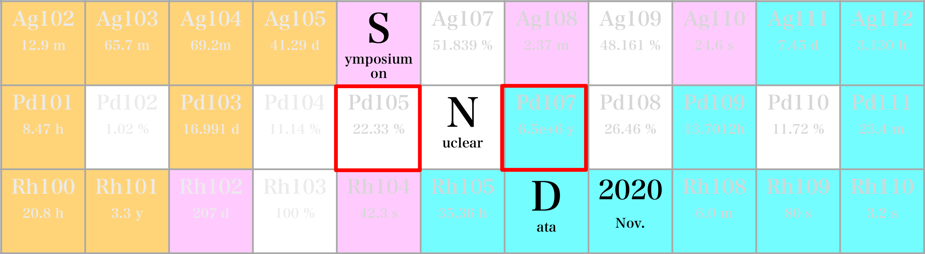#Symposium on Nuclear Data 2020

26-27 November 2020
RIKEN Wako Campus
Asia/Tokyo timezone

## Theoretical evaluation of non-resonant background strength in binary breakup reaction/二体分解反応における非共鳴バックグラウンドの理論的評価

26 Nov 2020, 16:55
1h 55m
RIBF201 (RIKEN Wako Campus)

### RIBF201

#### RIKEN Wako Campus

Hirosawa 2-1, Wako City, Saitama 351-0198, Japan
Poster Presentation

### Speaker

Mr Riu/履祐 Nakamoto/中本 (Department of Pure and Applied physics, Kansai University/関西大学　システム理工学部　物理・応用物理学科)

### Description

Inelastic scattering is useful tool to explore the nuclear structure in the excited states. In particular, the inelastic excitation to the continuum energy states above the particle decay threshold, which is often called breakup reaction, is very important because we can pin down a specific nuclear structure by controlling the exit channels, which are the combination of the emitted fragments.
A typical and good example about the inelastic scattering to the continuum can be seen in the breakup reaction of $^{12}$Be into the $\alpha$ + $^8$He and $^6$He + $^6$He channels. In this experiment, the careful multi-pole decomposition analysis (MDA) was performed, and the MDA analysis elucidates that many resonant states with a sharp width exist in the spins from $J^\pi=$ 0$^+$ to 8$^+$. The 0$^+$ resonances in the $\alpha$ + $^8$He channel appear in a close energy spacing of 0.5 MeV in the lower energy region below $E_{e.x.} \leq 15$ MeV, which is quite consistent to the energy scheme expected from the cluster resonances.
Basic and important quantities in the analysis of the resonance enhancement embedded in the continuum strength are the resonance parameters, such as the resonance energy and the decay width. In determining the resonance parameters, the evaluation of the non-resonant background strength is indispensable because the resonant enhancement, which has the strong energy dependence, are embedded in the non-resonant background contribution with a broad structure. Since the background strength is structure-less and must have the weak energy dependence, the shape of the non-resonant background strength is often assumed by the simple analytic function or evaluated from the simple reaction mechanism, such as the direct breakup without the final state interaction between the decaying fragments.
In this report, we investigate the structure of the non-resonant background continuum, which is generated by the binary breakup reaction, and explore the prescription to evaluate the background contribution by extending the Migdal-Watson formula for the s-wave breakup in the charge neutral system. In the calculation of the strength function for the binary breakup, we employ the complex scaling method (CSM), which is a powerful tool to describe the few-body continuum states. We handle the breakup reaction of $^{20}$Ne into $\alpha$ + $^{16}$O and $^{12}$Be into $\alpha$ + $^8$He. From the CSM calculation and the Migdal-Watoson theory, we propose the analytic function, which is appropriate to evaluate the background contribution for the binary breakup.

### Primary authors

Mr Riu/履祐 Nakamoto/中本 (Department of Pure and Applied physics, Kansai University/関西大学　システム理工学部　物理・応用物理学科) Dr Makoto/誠 Ito/伊藤 (Department of Pure and Applied physics, Kansai University/関西大学 システム理工学部 物理・応用物理学科) Dr Saito/齊藤 Akito/明登 (Department of Radiation Oncology, ) Prof. Shimoura/下浦 Susumu/享 (Center for Nuclear Study, School of Science, University of Tokyo)

### Presentation Materials

 SND2020_nakamoto.pptx commeted

Paper files: